Custom math worksheets at your fingertipsMath worksheet template "Binomic1"

This is not a ready-to-download math worksheet. It is a template, that is a preconfgured list of problems with parameters, that you can use to create your own math worksheet from. You can then change the resulting worksheet if some of the parameters need any tweaking. To use this template and create your own custom worksheet, you have to register first so you can edit worksheets. You can register and create an account in a minute - there is a free trial available. Register here, and you can use this template. There is no charge for joining and as a new member, you will get a free amount of starting credits. After registering, please choose the tab "My worksheets", then select "View templates".

Title

Binomic formulas: Expand and simplify

Description

This worksheet provides basic exercises to become familiar with the binomic formulas.

Elaboration

The first three tasks are assignment tasks, in which binomic formulas must be matched to their expanded form. The first two problems deal with the first two and then the third binomic formula. The third task then mixes all three formula types. This is followed by a problem in which the given binomial formulas must be multiplied out. Another two exercises are cloze texts in which binomial formulas are presented with their simplified forms and various elements such as arithmetic symbols, variables and numbers are to be added.Finally, the last task presents simplified terms that are to be converted into a binomic formula.

Preview

This is what the worksheet will look like. On the left hand side, the problem type is shown with its quickname. On the right, you will see a preview of the problem. This is just a sample, as dw-math will generate a new one when you load the template. Click on the underlined problem name to jump to the problem's detail page. You can download preview worksheets and solution sheets for this problem there. Note that all problem numbers are show as one here, in the worksheet they will be numbered sequentially. The worksheet's header and footer are not shown here.

 Match binomic formula to simplified formFind matching terms of original and simplified binomic formulas in two lists.Quickname: 5330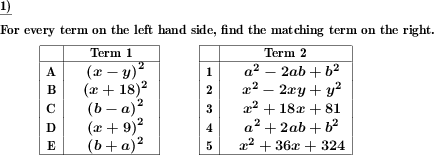Match binomic formula to simplified formFind matching terms of original and simplified binomic formulas in two lists.Quickname: 5330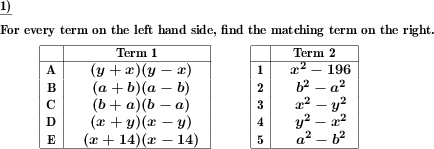Match binomic formula to simplified formFind matching terms of original and simplified binomic formulas in two lists.Quickname: 5330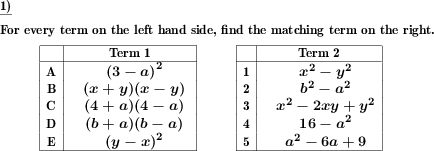Expand and simplify binomic formulaApply binomic formula expansion rules to simplify term. Quickname: 7813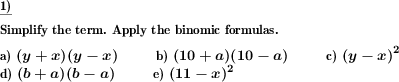Binomic formula fill in blanksFill gaps correctly in binomic formulasQuickname: 5815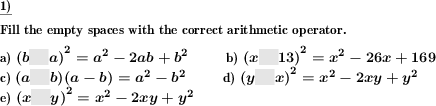Binomic formula fill in blanksFill gaps correctly in binomic formulasQuickname: 5815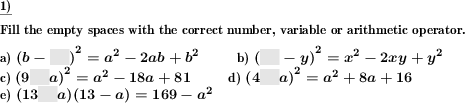Specify binomic formula for expanded termA term is given which is the expanded form of a binomic formula. The original binomic formula has to be found.Quickname: 6896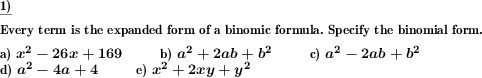These informational pages with samples describe math problems that can be combined on custom math worksheets with solutions for home and K-12 school use.
Deutsche Seiten
×